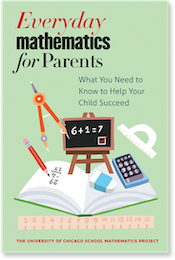## Understanding Everyday Mathematics

This section provides explanations for many of the common questions parents have about the Everyday Mathematics curriculum. Here you can learn more about the rationale behind Everyday Mathematics' position on topics like basic math facts and calculator use, in addition to tips on how to assist your child.

### Basic Facts

Helping children learn the basic facts is an important goal in the Everyday Mathematics curriculum. In this section, you can find out more about how the curriculum employs a variety of techniques to help children develop their "fact power", or basic number-fact reflexes.

### Computation

Everyday Mathematics recognizes that, even in the computer age, it is important to teach children how to compute "by hand". Here you can read more about how the curriculum provides all students with a variety of dependable and understandable methods of computation.

### Alternative Algorithms

Research has shown that teaching the standard U.S. algorithms for each of the four basic operations of arithmetic fails with large numbers of children, and that alternative algorithms are often easier for children to understand and learn. In this section, you can read more about how Everyday Mathematics introduces children to a variety of alternative procedures in addition to the customary algorithms.

### Calculator Use

In the Everyday Mathematics program, emphasis is placed on using the calculator as a tool for learning mathematics. You can read more here about how the use of calculators is incorporated to provide practice with place value and problem-solving skills in the curriculum.

### Meeting Individual Needs

Simply stated, the primary goal of Everyday Mathematics is to help more children learn more mathematics. This section explains how the curriculum expects higher levels of accomplishment at every grade level while also incorporating features that help make mathematics accessible to all students.

We believe it is very important to help parents become actively involved in their child's mathematical education. Here you can see some suggestions for how you can learn about the mathematics your child is studying in school, and how you can help reinforce their math learning at home.The University of Chicago School Mathematics Project

University of Chicago Press